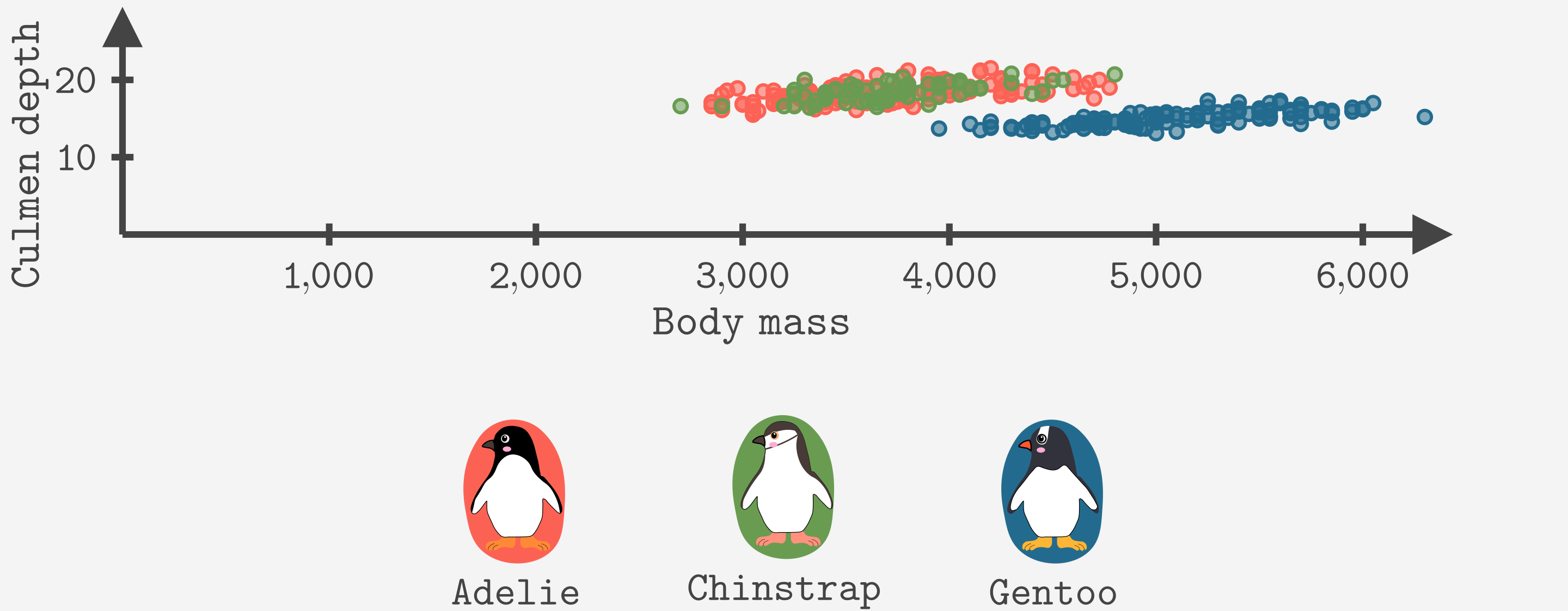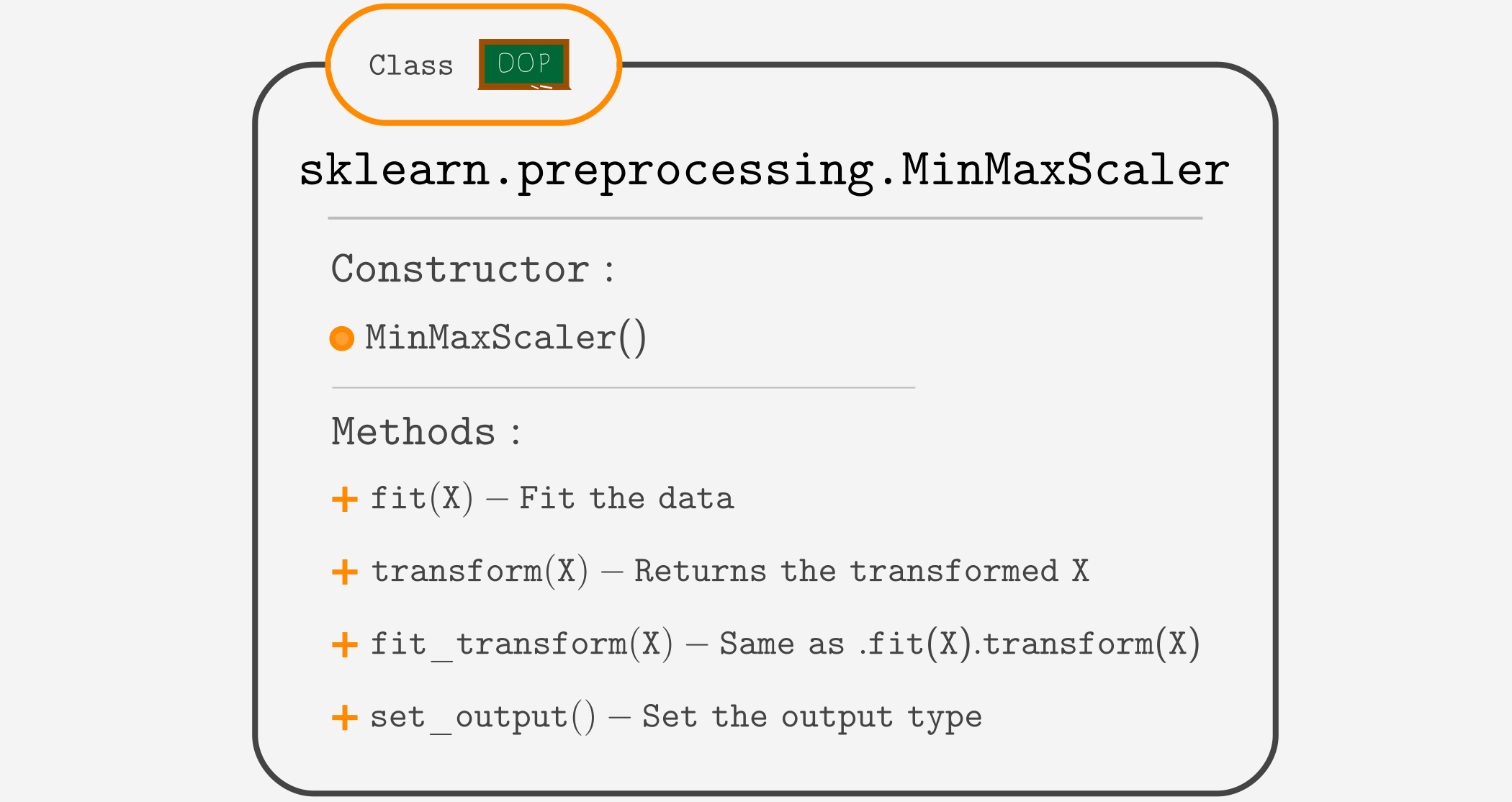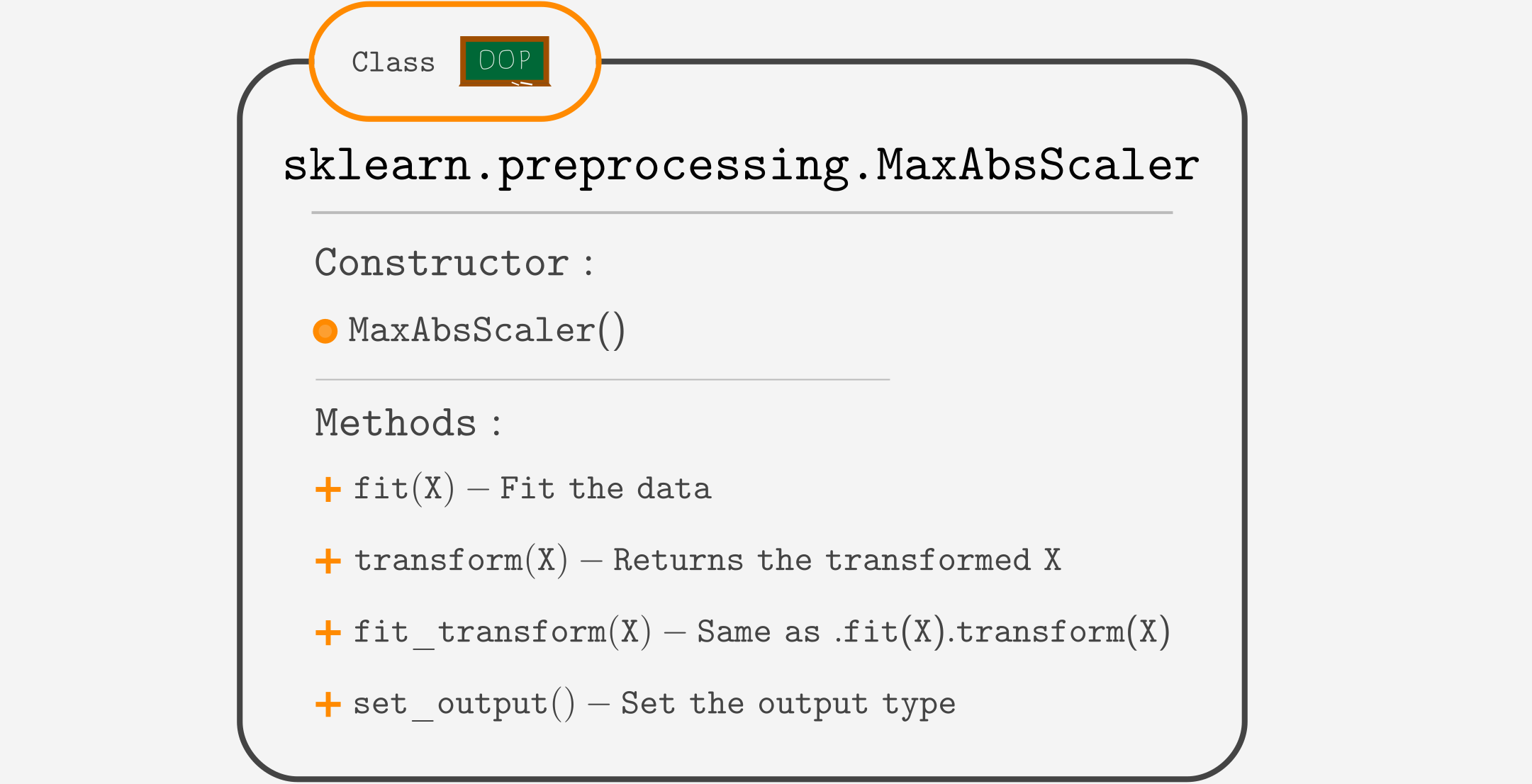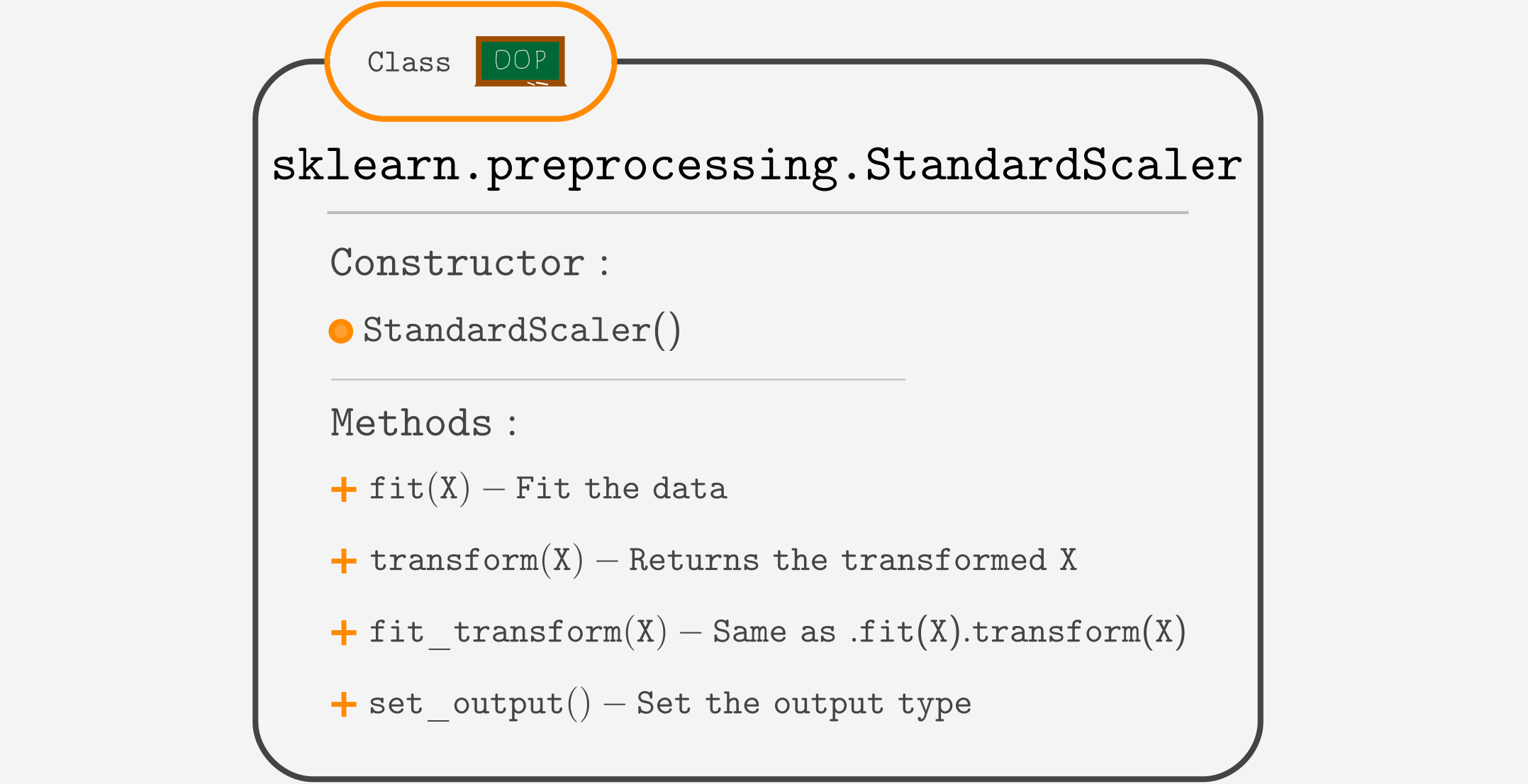Course Content

ML Introduction with scikit-learn

##StandardScaler, MinMaxScaler, MaxAbsScaler

There are three popular approaches to scaling the data:

• MinMaxScaler - scales features to a [0, 1] range.
• MaxAbsScaler – scales features such as the maximum absolute value is 1 (so the data is guaranteed to be in a [-1, 1] range).
• StandardScaler – standardize features making the mean equal to 0 and variance equal to 1.

To demonstrate how the scalers work, we will use the `'culmen_depth_mm'` and `'body_mass_g'` features of the Penguins dataset. Let's plot them.### MinMaxScaler

The `MinMaxScaler` works by subtracting the minimum value (to make values start from zero) and then dividing by (x_max - x_min) to make it less or equal to 1.Here is the video showing how `MinMaxScaler` works:

### MaxAbsScaler

The `MaxAbsScaler` works by finding the maximum absolute value and dividing each value by it. This ensures that the maximum absolute value is 1.Here is the video showing how `MaxAbsScaler` works:

### StandardScaler

The idea of `StandardScaler` comes from statistics. It works by subtracting the mean (to center around zero) and dividing by the standard deviation (to make the variance equal to 1).

Note

If you do not understand what the mean, standard deviation, and variance are, you can check our Learning Statistics with Python course.
However, this knowledge is not mandatory to move on.Here is a video showing how `StandardScaler` works.

Let's look at the coding example using `MinMaxScaler` (other scalers are used exactly the same).The output is not the prettiest since scalers transform the data to a NumPy array, but with pipelines, it won't be a problem.

Note

You should only scale the feature columns (the `X` variable). No need to scale the target. It will only make it harder to get an inverse transform.

### Which scaler to use?

A `StandardScaler` is less sensitive to outliers, so it is a good default scaler.
If you don't like the `StandardScaler`, between `MinMaxScaler` and `MaxAbsScaler`, it comes down to personal preferences, scaling data to the [0,1] range or to [-1,1].

Everything was clear?

Section 2. Chapter 10